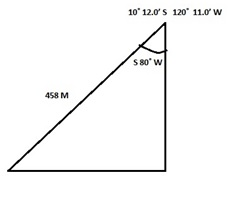# EXERCISE 1– PLANE AND PARALLEL SAILING (Numerical Solution)

1. ###### COURSE = 260˚ ,  T = S 80˚ W ,  Dist. = 458 MWe know that:
D’Lat = (Dist. × Cos Co.)
= 458 × Cos 80˚
=  79.5’
= 1˚ 19’ S

Lat left            = 10˚ 12.0’ S
D’LAT              = 01˚ 19.0’ S
LAT ARR’D      = 11˚ 31.0’ S

So,  M’ LAT            = 10˚ 51.5’ S

DEP. = (D’LAT × Tan Co.)
= 450.86’

D’LONG =( DEP./Cos M’LAT)
= 459.08’ W OR 007˚ 39’ W

LONG LEFT                               = 120˚ 11.0’ W
LONG ARR’D                            = 127˚ 50.0’ W

1. ###### COURSE = 340˚, T= N 20˚ W , Dist. = 510 MWe know that:

D’Lat = (Dist. × Cos Co.)
= (510 × Cos 20˚)
= 479.24’
= 7˚ 59.2’ N

Lat left            = 00˚ 10.0’ S
D’LAT              = 07˚ 59.2’ N
LAT ARR’D      = 07˚ 49.2’ N

So, M’ LAT            = 03˚ 49.6’ N

DEP. =( D’LAT × Tan Co.)
= 174.42’

D’LONG = (DEP./Cos M’LAT)
= 174.42’ E OR 002˚ 54.4’ W

LONG LEFT                               = 179˚ 40.0’ W
LONG ARR’D                            = 177˚ 25.2’ E#### Vikrant_sharma

•Niranjan nar Shandilya says:

Sir problem in stability chapter 3 ques no 4 is not correctely solve kindly correct all these types of error

•Krish says:

What is the mistake

•JeffReyes says:

I think some of your Dlong Computations are incorrect

•Ukeme Hillary Eboh says: